Courses

# Metal Cutting And Tool Materials - 1

## 10 Questions MCQ Test Topicwise Question Bank for GATE Mechanical Engineering | Metal Cutting And Tool Materials - 1

Description
This mock test of Metal Cutting And Tool Materials - 1 for Mechanical Engineering helps you for every Mechanical Engineering entrance exam. This contains 10 Multiple Choice Questions for Mechanical Engineering Metal Cutting And Tool Materials - 1 (mcq) to study with solutions a complete question bank. The solved questions answers in this Metal Cutting And Tool Materials - 1 quiz give you a good mix of easy questions and tough questions. Mechanical Engineering students definitely take this Metal Cutting And Tool Materials - 1 exercise for a better result in the exam. You can find other Metal Cutting And Tool Materials - 1 extra questions, long questions & short questions for Mechanical Engineering on EduRev as well by searching above.
QUESTION: 1

### In an orthogonal cutting process, rake angle of the tool is 220 and friction angle is 260. Using Merchant’s shear angle relationship, the value of shear angle will be

Solution:

As per Merchant’s analysis: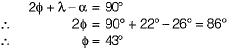QUESTION: 2

### During an orthogonal machining operation on mild steel, the results obtained are: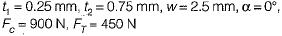What is the coefficient of friction between the tool and the chip?

Solution: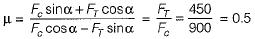QUESTION: 3

### Match the List-I (Cutting tool material!) with List-II (Value of ‘n’ in equation, VTn = C):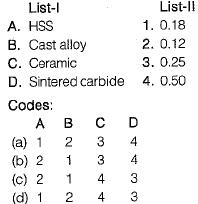Solution:
QUESTION: 4

The approximate value of the tool life exponent 'n’ of cemented carbide tool is

Solution: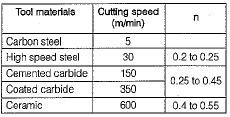QUESTION: 5

Which of the following work material has highest machining constant?

Solution: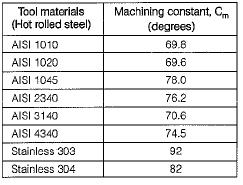QUESTION: 6

In an orthogonal cutting process, rake angle of the tool is 200 and friction angle is 240. Using Lee's and Shaffer's shear angle, the value of shear angle will be

Solution: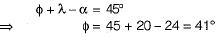QUESTION: 7

Using the Taylor equation for tool life and letting n = 0.5 and C= 400, calculate the percentage increase in tool life when the cutting speed is reduced by 50%.

Solution: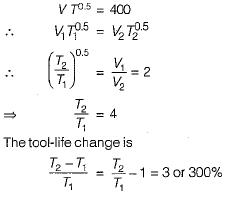QUESTION: 8

In material removal process, chipping is used to describe

Solution:
QUESTION: 9

Which isotherms have severe temperature gradient of the following temperature distribution in the cutting zone?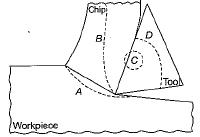Solution:

Severe temperature exists at the tool-chip interface.

QUESTION: 10

Optimum cutting speed for minimum cost (Vcmin) and optimum cutting speed for maximum production rate (Vr max) have which one of the following relationship?

Solution:

Minimum cost implies for minimum tool wear. Maximum production rate means higher speed irrespective of tool wear.
∴ Vr max < Vc min